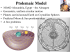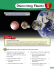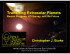# Spring 2015-ASTR 201 Homework #6 solutions

## Transcription

Spring 2015-ASTR 201 Homework #6 solutions
```Spring 2015-ASTR 201 Homework #6 solutions
Chapter 13
11) How do the orbits of known extrasolar planets differ from those of planets in our solar
system? Why are these orbits surprising?
Solution:
1. Many of the known extrasolar planets orbit their stars more closely than Mercury orbits
the Sun and non are located as far from their stars as the Jovian planets of our solar
system.
2. Many of these planets also have large orbital eccentricities, telling us that their elliptical
orbits have very stretched-out shapes.
These orbits don’t hold with the predictions of nebular theory: Jovian planets form as
gravity pulls in gas around large, icy planetesimals that accrete in a spinning disk of material
around a young star. The theory predicts that Jovian planets should form only in the cold
outer regions of star systems (because it must be cold for ice to condense) and these
planets should be born with nearly circular orbits (matching the orderly, circular motion of
the spinning disk). The many known extrasolar planets that appear Jovian in nature but
have close-in or highly elliptical orbits present a direct challenge to these ideas. The
surprising orbits off many known extrasolar planets have caused scientists to reexamine the
nebular theory of solar system formation.
44) Comparing Methods. What are the strengths and limitations of the Doppler and transit
techniques? What kinds of planets are easiest to detect with each method? Are there certain
planets that each cannot detect, even if the planets are very large? Explain. What advantages
are gained if a planet can be detected by both methods?
Solution: (See Table 13.1 on page 374)
Doppler Technique:
Strengths: Current techniques can measure a star’s velocity to within ~1 m/s (walking
speed) which corresponds to a Doppler shift of just 0.0000003%. This method can find
planets that exert a considerably small gravitational tug or gravitational tugs of more than
one planet on a single star. This method also detects planets in all orbit orientations except
for face-on with respect to Earth.
Limitations: Searching for gravitational effects from orbiting planets means it is better for
finding massive planets (like Jupiter) than smaller planets (like Earth). It is best suited for
finding massive planets orbiting relatively close to their star, because being closer creates a
stronger gravitational tug. Planets farther from their stars are more difficult to detect using
this method. The technique requires observing long enough to see a star’s orbit around its
center of mass, so it is biased towards finding close-in planets with short orbital periods. It
also requires a fairly large telescope to obtain spectra with high enough resolution in order
to find very small Doppler shifts. When the orbital inclination with respect to Earth is
unknown, the method provides only minimum mass for the planets.
Transit Technique:
Strengths: The technique allows many stars to be observed at once and can reveal planets
far smaller than ones found with astrometric or Doppler techniques. Small planets create
very little, but measureable, dimming of the star’s brightness while creating very little
gravitational tug. In a multiplanet system, a small planet leaves a distinct transit signature
because plants with different periods will rarely transit in front of a star at the same time.
The transit technique can detect small planets in such a system while other techniques are
not as reliable. In some cases, transits can be measured with small personal telescopes (an
extrasolar planet has been detected with a telescope as small as 4 inches in diameter).
Some atmospheric information can be provided in the case of a measurable eclipse.
Limitations: Most stars exhibit (random) variations in brightness, so we can be confident
that a planet is detected only if the observed dips in brightness repeat with a regular period
(indicating that the same planet is passing in front of the star with each orbit). This
limitation in the technique requires monitoring a star’s brightness for at least three full
orbits of the planet. The planet’s orbit needs to be aligned “just right” (edge-on orbit) so
that we observe as it passes in front of their star as seen from Earth; so the method only
works for a small fraction of planetary systems. The method is biased towards close-in,
larger planets with short orbital periods because less observing time is needed for repeated
transits and larger planets create larger dips in brightness as they block out more light. Only
space observatories have the sensitivity needed to detect small planets.
Both Techniques:
If a planet can be detected by both techniques, it is easier to confirm as an extrasolar
planet. The transit method gives the planet’s radius while the Doppler method gives the
mass; both methods can give enough information to calculate the density of the planet.
49) Lost in the Glare. How hard would it be for an alien astronomer to detect the light from
planets in our solar system compared to the light from the Sun itself?
a. Calculate the fraction of the total emitted sunlight that is reflected by Earth. (Hint: Imagine a
sphere around the Sun the size of the planet’s orbit (area = 42 ). What fraction of that area
2
does the disk of a planet take up (area = ℎ
)? Earth’s reflectivity is 29%).
b. Would detecting Jupiter be easier or harder than detecting Earth? Comment on whether you
think Jupiter’s larger size or greater distance has a stronger effect on its detectability. You may
neglect any difference in reflectivity between Earth and Jupiter.
Solution:
a. (See Mathematical Insight 13.3 on page 384)
The fraction of total emitted sunlight reflected by Earth can be calculated using the
formulas given in the hint. Imagine the sphere as described above and take a thin slice
(cross section) of it. The area swept by Earth’s orbit would be the blue circle in the
image below. The area blocked by Earth itself is the green circle.
Area swept by Earth’s orbit
Area blocked by Earth
r
a
2
Area of Earth′ s disk
ℎ
=
Area of planet’s orbit
42
(6371 km)2
=
= 4.5 × 10−10
4 × (1.5 × 108 km)2
This is the fraction that would be completely blocked if the planet were opaque (did not
reflect any light). Use Earth’s reflectivity to calculate the fraction of total emitted
sunlight reflected by Earth:
(4.5 × 10−10 ) × 0.29 = .  × − = .  (a very small amount)
b. Jupiter’s larger size would be easier than Earth for detection via the Doppler or Transit
techniques, but since it orbits at a greater distance which would reduce the chances of
detection. Jupiter has a relatively long orbital period (~12 years), so in order to see 3
repeated transits for the Transit method, alien astronomers would need to observe for
at least 36 years. Earth’s small size might prove difficult to detect; however, using the
Transit method, alien astronomers would only need to observe for 3 years to see 3
repeated transits. Because of the shorter orbital period, Earth would be easier than
Jupiter to detect with an alien version of the Kepler mission.
The gravitational effects due to the Jupiter’s large mass would be somewhat negated by
its distance from the Sun, making detection via the Doppler method difficult (but not
impossible). Earth’s small mass would make it extremely difficult to detect with this
method.
53) Finding Orbit Sizes. The Doppler method allows us to find a planet’s semimajor axis using
just the orbital period and the star’s mass (See Mathematical Insight 13.1 on page 375).
a. Imagine that a new planet is discovered orbiting a 2 MSun star with a period of 5 days. What is
its semimajor axis?
b. Another planet is discovered orbiting a 0.5 MSun star with a period of 100 days. What is its
semimajor axis?
Solution:
a. Mstar = 2 × MSun = 2 × (2 × 1030 kg) = 4 × 1030 kg
24 hours 3600 sec
P = 5 days ×
×
= 432000 seconds
1 day
1 hour
3
−11
(6.67 × 10
GMstar 2
√
√
a=
p
=
4π2 planet
3
m3
) × (4 × 1030 kg) × (432000 s)2
kg × s
4π2
≈  ×
b. Mstar = 0.5 × MSun = 0.5 × (2 × 1030 kg) = 1 × 1030 kg
24 hours 3600 sec
P = 100 days ×
×
= 8.64 × 106 seconds
1 day
1 hour
3
3 GM
√
star 2
a= √
pplanet =
2
4π
(6.67 × 10−11
m3
) × (1 × 1030 kg) × (8.64 × 106 s)2
kg × s
4π2
≈  ×
Chapter 14
6) Why does nuclear fusion require high temperatures and pressures?
Solution:
Nuclear fusion requires high temperatures because the nuclei must collide at very high
speeds if they are to come close enough together to fuse. High temperatures mean the
particles are moving faster, so the collisions are harder, making fusion reactions more likely.
High pressure is necessary because, without it, the hot plasma of the solar core would
simply explode into space, shutting off nuclear reactions.
7) What is the overall nuclear fusion reaction in the Sun? Briefly describe the proton-proton
chain.
Solution:
The Sun releases energy by fusing four hydrogen nuclei into one helium nucleus. The
proton-proton chain involves 3 steps:
1. Two protons fuse to form a deuterium nucleus (an isotope of hydrogen), a positron, and
a neutrino. This step occurs twice in the overall reaction, which requires a total of four
protons.
2. A deuterium nuclei collides and fuses with a proton, creating a nucleus of Helium-3 (a
rare form of helium with two protons and one neutron) and a gamma-ray photon. This
step also occurs twice in the overall reaction.
3. Another neutron is added to the Helium-3, creating Helium-4 (2 protons and 2 neutrons)
and two excess protons. This can happen in different ways, but the most common is a
collision of two Helium-3 nuclei.
9) Why has the Sun gradually brightened with time?
Solution:
The processes involved in gravitational equilibrium prevent erratic changes in the fusion
rate and ensure that the fusion rate gradually rises over billions of years. This rise explains
the gradual brightening of the Sun with time. Each fusion reaction converts four hydrogen
nuclei into one helium nucleus, causing the total number of independent particles in the
core is gradually decreasing with time, which causes the solar core to shrink. This slow
shrinkage gradually increases the core temperature and fusion rate, keeping the core
pressure high enough to counteract the stronger compression of gravity. Theoretical
models indicate the Sun’s c ore temperature should have increased enough to raise its
fusion rate and luminosity by ~30% since the Sun was born 4.5 billion years ago.
Extra credit
50) The Lifetime of the Sun. The total mass of the Sun is about 2 × 1030 kg, of which about 70%
was hydrogen when the Sun formed. However, only about 13% of this hydrogen ever becomes
available for fusion in the core. The rest remains in layers of the Sun where the temperature is
too low for fusion.
a. Use the given data to calculate the total mass of hydrogen available for fusion over the
b. The Sun fuses about 600 billion kg of hydrogen each second. Based on your result from part
a, calculate how long the Sun’s initial supply of hydrogen can last. Give your answer in both
seconds and years.
c. Given that our solar system is now about 4.6 billion years old, when will we need to worry
about the Sun running out of hydrogen for fusion?
Solution:
a. The total mass of hydrogen available for fusion over the lifetime of the Sun depends on
how much hydrogen was available when the Sun formed. Calculate the amount of
hydrogen (in kg) in the Sun and then the amount available for fusion:
MSun × (% of Hydrogen at formation) × (% available for fusion)
= (2 × 1030 kg) × (0.70) × (0.13)
= .  ×
b. The Sun’s initial supply of hydrogen can last for
1.82 × 1029 kg available for fusion
1.82 × 1029 kg
=
≈  ×
600 billion kg fused / second
600 × 109 kg/s
1 hour
1 day
1 year
3 × 1017 seconds ×
×
×
= 9.62 × 109 years
3600 s 24 hours 365 days
≈ .
c. We will need to worry about the Sun running out of hydrogen for fusion in
9.6 billion years − 4.6 billion years ≈
```

### planet dance.pages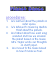### Matthew`s game - Parkfield Primary School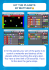### Friday 25th October 2013 4.00 p.m. Professor Linda T. Elkins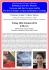### The 9 Planets - Curriculum for Excellence Science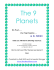### The search for extraterrestrial unintelligence Par Jonathan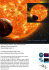### Be Chart Smart Name: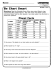### Bodies of our Solar System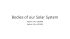### Ptolemaic Model